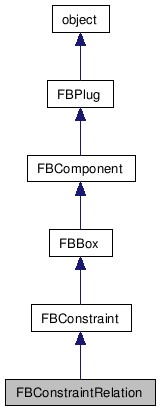# FBConstraintRelation Class Reference

FBConstraintRelation FBConstraintRelation FBConstraintRelation FBConstraintRelation FBConstraintRelation FBConstraintRelation FBConstraintRelation FBConstraintRelation FBConstraintRelation FBConstraintRelation FBConstraintRelation FBConstraintRelation FBConstraintRelation FBConstraintRelation FBConstraintRelation FBConstraintRelation FBConstraintRelation FBConstraintRelation FBConstraintRelation FBConstraintRelation FBConstraintRelation FBConstraintRelation FBConstraintRelation FBConstraintRelation FBConstraintRelation FBConstraintRelation FBConstraintRelation FBConstraintRelation FBConstraintRelation FBConstraintRelation FBConstraintRelation FBConstraintRelation FBConstraintRelation FBConstraintRelation FBConstraintRelation FBConstraintRelation FBConstraintRelation FBConstraintRelation FBConstraintRelation FBConstraintRelation FBConstraintRelation FBConstraintRelation FBConstraintRelation FBConstraintRelation FBConstraintRelation FBConstraintRelation FBConstraintRelation FBConstraintRelation FBConstraintRelation FBConstraintRelation FBConstraintRelation FBConstraintRelation FBConstraintRelation FBConstraintRelation FBConstraintRelation FBConstraintRelation FBConstraintRelation FBConstraintRelation FBConstraintRelation FBConstraintRelation FBConstraintRelation FBConstraintRelation FBConstraintRelation FBConstraintRelation FBConstraintRelation FBConstraintRelation FBConstraintRelation FBConstraintRelation FBConstraintRelation FBConstraintRelation FBConstraintRelation FBConstraintRelation FBConstraintRelation FBConstraintRelation FBConstraintRelation FBConstraintRelation FBConstraintRelation FBConstraintRelation FBConstraintRelation FBConstraintRelation FBConstraintRelation FBConstraintRelation FBConstraintRelation FBConstraintRelation FBConstraintRelation FBConstraintRelation FBConstraintRelation FBConstraintRelation FBConstraintRelation FBConstraintRelation FBConstraintRelation FBConstraintRelation FBConstraintRelation FBConstraintRelation FBConstraintRelation FBConstraintRelation FBConstraintRelation FBConstraintRelation FBConstraintRelation FBConstraintRelation FBConstraintRelation FBConstraintRelation FBConstraintRelation FBConstraintRelation FBConstraintRelation FBConstraintRelation FBConstraintRelation FBConstraintRelation FBConstraintRelation FBConstraintRelation FBConstraintRelation FBConstraintRelation FBConstraintRelation FBConstraintRelation FBConstraintRelation FBConstraintRelation FBConstraintRelation FBConstraintRelation FBConstraintRelation FBConstraintRelation
Inheritance diagram for FBConstraintRelation:[legend]

List of all members.

## Detailed Description

ConstraintRelation class.

This class exposes the relation constraint and allows addition of new boxes and removal of existing ones.

## Public Member Functions

tuple< bool, int, int GetBoxPosition ()
Get a box position in the GUI.
FBConstraintRelation (str pName)
Constructor.
FBBox  ConstrainObject (FBBox pConstrainedObject)
FBBox  CreateFunctionBox (str pGroup, str pName)
Create a function box.
FBBox  SetAsSource (FBBox pSource)
Create a sender box.
bool  SetBoxPosition (FBBox pBox, int pX, int pY)
Set a box position in the GUI.

## Public Attributes

FBListBox  Boxes
List: Boxes used in this constraint.

## Constructor & Destructor Documentation

 FBConstraintRelation ( str pName )

Constructor.

Parameters:
 pName Name of constraint.

## Member Function Documentation

 tuple GetBoxPosition ( )

Get a box position in the GUI.

Get the position of a box within the constraint layout view.

Returns:
A tuple containing: the result of operation (bool), X value (int), and Y value(int)

 FBBox ConstrainObject ( FBBox pConstrainedObject )

Use an existing FBBox object to create a receiver in the relation.

Parameters:
 pConstrainedObject Destination box to insert in the constraint.
Returns:
A place holder box for the object.

 FBBox CreateFunctionBox ( str pGroup, str pName )

Create a function box.

Ask the constraint to create new function box.

Parameters:
 pGroup Name of the group under which the function is located in the Constraint Relation GUI (case-sensitive!). pName Name of the function, as seen in the GUI (case-sensitive!).
Returns:
The newly created function box, or NULL if the name/group combination was invalid.

 FBBox SetAsSource ( FBBox pSource )

Create a sender box.

Use an existing FBBox object to create a sender in the relation.

Parameters:
 pSource Source box to insert in the constraint.
Returns:
A place holder box for the object.

 bool SetBoxPosition ( FBBox pBox, int pX, int pY )

Set a box position in the GUI.

Set the position of a box within the constraint layout view.

Parameters:
 pBox Box which needs to be moved. pX New X position. pY New Y position.
Returns:
A boolean value indicating success (True) or failure (False).

## Member Data Documentation

 FBListBox Boxes

List: Boxes used in this constraint.

Generated on Tue Feb 9 19:34:01 2010 for Python Reference Guide by1.5.3